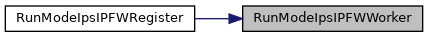suricata
runmode-ipfw.h File Reference
This graph shows which files directly or indirectly include this file: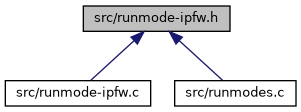Go to the source code of this file.

Functions

int RunModeIpsIPFWAutoFp (void)

int RunModeIpsIPFWWorker (void)

void RunModeIpsIPFWRegister (void)

const char * RunModeIpsIPFWGetDefaultMode (void)

Function Documentation

 int RunModeIpsIPFWAutoFp ( void )

Definition at line 66 of file runmode-ipfw.c.

Referenced by RunModeIpsIPFWRegister().

Here is the call graph for this function: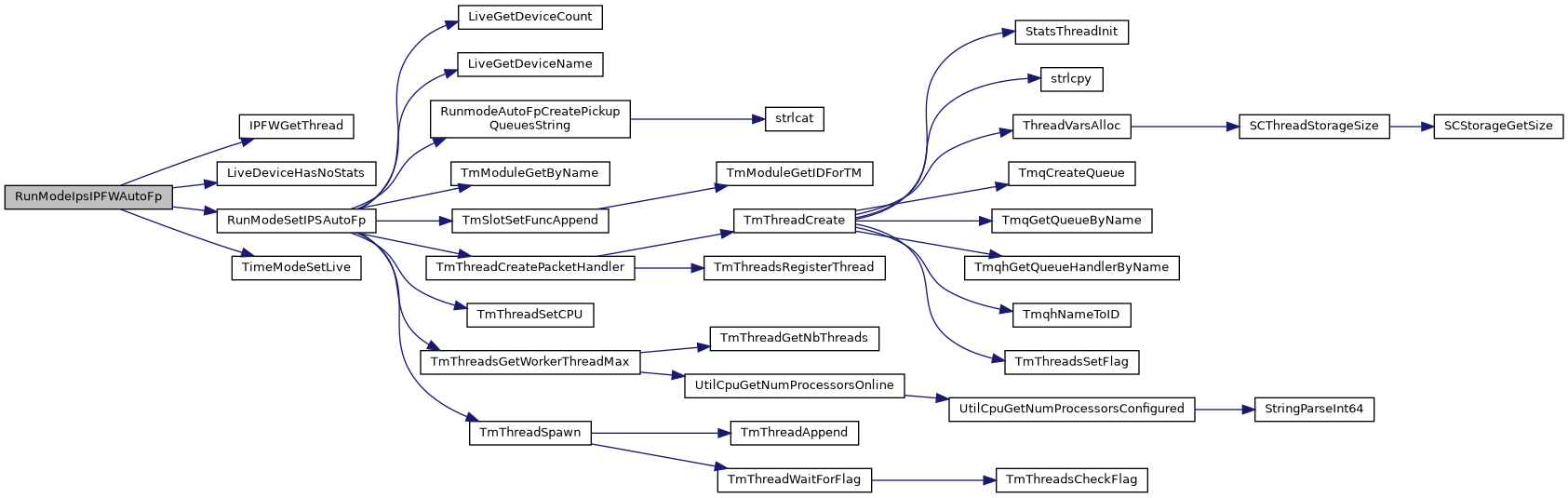Here is the caller graph for this function: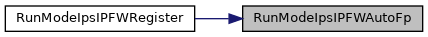const char* RunModeIpsIPFWGetDefaultMode ( void )

Definition at line 46 of file runmode-ipfw.c.

Referenced by RunModeDispatch().

Here is the caller graph for this function:void RunModeIpsIPFWRegister ( void )

Definition at line 51 of file runmode-ipfw.c.

Referenced by RunModeRegisterRunModes().

Here is the call graph for this function: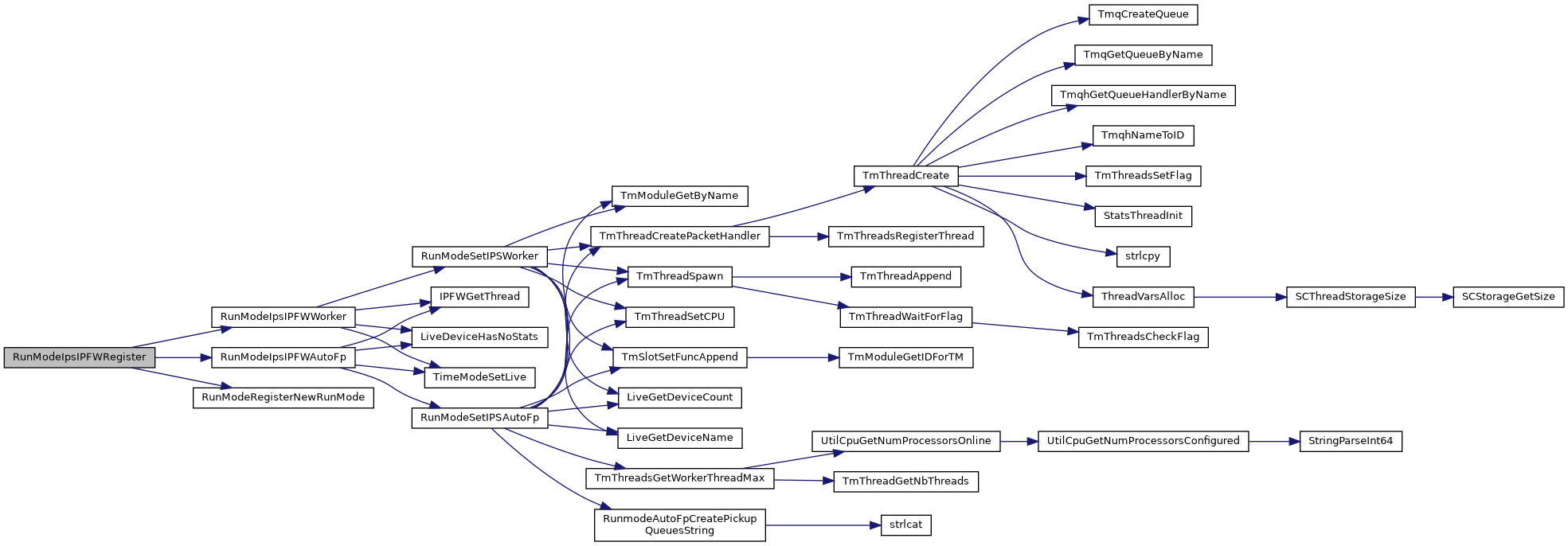Here is the caller graph for this function:int RunModeIpsIPFWWorker ( void )

Definition at line 86 of file runmode-ipfw.c.

Referenced by RunModeIpsIPFWRegister().

Here is the call graph for this function: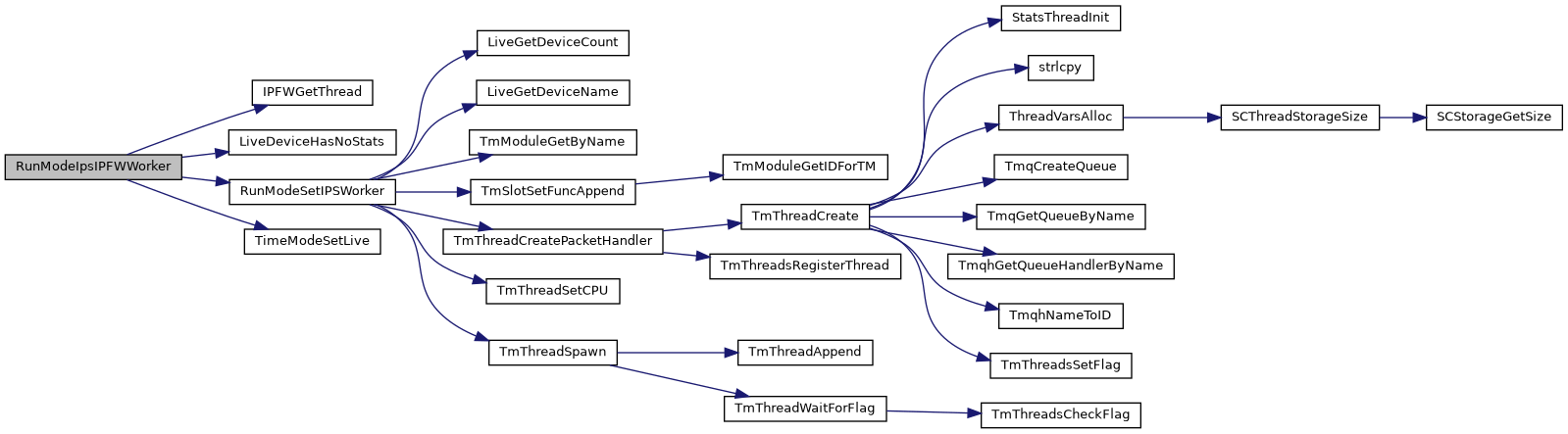Here is the caller graph for this function: